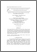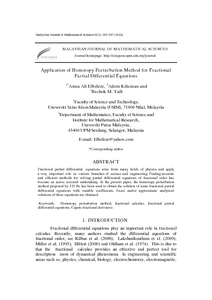# Application of homotopy perturbation method for fractional partial differential equations

## Citation

Elbeleze, Asma Ali and Kilicman, Adem and M. Taib, Bachok (2014) Application of homotopy perturbation method for fractional partial differential equations. Malaysian Journal of Mathematical Sciences, 8 (2). pp. 265-287. ISSN 1823-8343; ESSN: 2289-750X

## Abstract

Fractional partial differential equations arise from many fields of physics and apply a very important role in various branches of science and engineering. Finding accurate and efficient methods for solving partial differential equations of fractional order has become an active research undertaking. In the present paper, the homotopy perturbation method proposed by J-H He has been used to obtain the solution of some fractional partial differential equations with variable coefficients. Exact and/or approximate analytical solutions of these equations are obtained.Preview
PDF
38948.pdfView Item